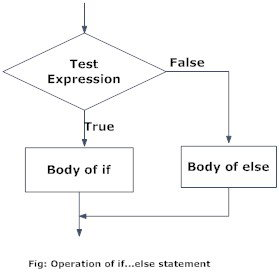# Python if else Statement Programs

in this post you will learn how to use Python if else Statement Programs using different forms of if..else statement.

## What are if…else statement in Python?

Decision making is required for execute a code only if a certain condition is true.

The `if…elif…else` statement is used in Python for decision making.

### Python if Statement Syntax

```if test expression:
statement(s)
```

Here, the program evaluates the `test expression` and will execute the statement(s) only if the test expression is `True` means satisfied the equation.

If the text expression is `False` means equation is not satisfied, the statement(s) is not executed.

In Python, the body of the `if` statement is indicated by the indentation. Body starts with an indentation and the first unindented line marks the end.

Python interprets non-zero values as `True``None` and `0` are interpreted as `False`.

### Python if Statement Flowchart### Example: Python if Statement

```# If the number is positive, print an appropriate result

num = 3
if num > 0:
print(num, "is a positive number.")
print("This is always printed.")

num = -1
if num > 0:
print(num, "is a positive number.")
print("This is also always printed.")
```

```3 is a positive number
This is always printed
This is also always printed.```

In the above example, `num > 0` is the test expression.

The body of `if` is executed only if this evaluates to `True`.

When variable num is equal to 3, test expression is true and body inside body of `if` is executed.

If variable num is equal to -1, test expression is false and body inside body of `if` is skipped.

The `print()` statement falls outside of the `if` block (unindented). Hence, it is executed regardless of the test expression.

## Python if…else Statement

### Syntax of if…else

```if test expression:
Body of if
else:
Body of else
```

The `if..else` statement evaluates `test expression` and will execute body of `if` only when test condition is `True`.

If the condition is `False`, body of `else` is executed. Indentation is used to separate the blocks.

### Python if..else Flowchart### Example of if…else

```# Program checks if the number is positive or negative
# And displays an appropriate message

num = 3

# Try these two variations as well.
# num = -5
# num = 0

if num >= 0:
print("Positive or Zero")
else:
print("Negative number")
```

In the above example, when num is equal to 3, the test expression is true and body of `if` is executed and `body` of else is skipped.

If num is equal to -5, the test expression is false and body of `else` is executed and body of `if`is skipped.

If num is equal to 0, the test expression is true and body of `if` is executed and `body` of else is skipped.

## Python if…elif…else Statement

### Syntax of if…elif…else

```if test expression:
Body of if
elif test expression:
Body of elif
else:
Body of else
```

The `elif` is shortform for else if in python.  elif helps to check for multiple expressions.

If the condition for `if` is `False`, it checks the condition of the next `elif` block and so on.

If all the conditions are `False`, body of else is executed.

Only one block among the several `if...elif...else` blocks is executed according to the condition.

The `if` block can have only one `else` block. But it can have multiple `elif` blocks.

### Flowchart of if…elif…else### Example of if…elif…else

```# In this program,
# we check if the number is positive or
# negative or zero and
# display an appropriate message

num = 3.4

# Try these two variations as well:
# num = 0
# num = -4.5

if num > 0:
print("Positive number")
elif num == 0:
print("Zero")
else:
print("Negative number")```

When variable num is positive, Positive number is printed.

If num is equal to 0, Zero is printed.

If num is negative, Negative number is printed

## Python Nested if statements

We can have a `if...elif...else` statement inside another `if...elif...else` statement. This is called nesting in computer programming.

Any number of these statements can be nested inside one another. Indentation is the only way to figure out the level of nesting. This can get confusing, so must be avoided if we can.

### Python Nested if Example

``````# In this program, we input a number
# check if the number is positive or
# negative or zero and display
# an appropriate message
# This time we use nested if

num = float(input("Enter a number: "))
if num >= 0:
if num == 0:
print("Zero")
else:
print("Positive number")
else:
print("Negative number")``````

Output 1

```Enter a number: 5
Positive number
```

Output 2

```Enter a number: -1
Negative number
```

Output 3

```Enter a number: 0
Zero```